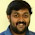## Pages

SUBSCRIBE HERE TO GET POST UPDATES VIA EMAIL :

Delivered by FeedBurner

# while, do while Loops - Basic Java Tutorials For Selenium WebDriver

As we have learnt in my previous post, loops(for loop, while loop) in java software development language or any other software programming languages are useful to execute block of code multiple times. You will have to use loops in your selenium webdriver software tests very frequently. We have already learnt for loop with different examples in my previous post. Now let me describe you while loop and do while loop with practical examples in java software development language.

while Loop
Block of code which is written inside while loop will be executed till the condition of while loop remains true.
Example :
``````        int i = 0;
while(i<=3){
System.out.println("Value Of Variable i Is "+i);
i++;
}``````
In above given example of  java software development language, while loop will be executed four times.

do while Loop
Same as while loop, do while loop will be executed till the condition returns true.
Example :
`````` int j=0;
do{
System.out.println("Value Of Variable j Is "+j);
j=j-1;
}while(j>0);``````
In above given example, while loop will be executed only one time.

Difference between while and do while loop
There is one difference between while and do while loop.
• while loop will check condition at the beginning of code block so It will be executed only if condition (while(i<=3)) returns true.
• do while loop will check condition at the end of code block so It will be executed minimum one time. After 1st time execution, it will check the condition and if it returns true then code of block will be executed once more or multiple time.
Disadvantage of while or do while loop
If you will forget to Increment or decrements variable value inside while loop block then block of code will be executed infinite time.
Example :
``````        int i = 0;
while(i<=3){
System.out.println("Value Of Variable i Is "+i);
}``````
Above given while loop will be executed infinite time because variable is not incremented inside while loop block.

Bellow given full example of while and do while loops will clear out your all doubts. Simple run it in your eclipse and verify result.
``````public class Whileloop {

public static void main(String[] args) {

//while loop - will be executed till condition returns true.
System.out.println("***while loop example***");
int i = 0; //Variable initialization
while(i<=3){
System.out.println("Value Of Variable i Is "+i);
i++;//Incrementing value of i by 1.
}

//do while loop - will be executed minimum one time without considering condition.
System.out.println("");
System.out.println("***do while loop example***");
int j=3; //Variable initialization
do{
System.out.println("Value Of Variable j Is "+j);
j=j-1;//Decrementing value of j by 1;
}while(j>=0);
}
}``````

Output of above given example will be as bellow.
``````***while loop example***
Value Of Variable i Is 0
Value Of Variable i Is 1
Value Of Variable i Is 2
Value Of Variable i Is 3

***do while loop example***
Value Of Variable j Is 3
Value Of Variable j Is 2
Value Of Variable j Is 1
Value Of Variable j Is 0``````

1.do while Loop
Same as while loop, do while loop will be executed till the condition returns true.
Example :
int j=0;
do{
System.out.println("Value Of Variable j Is "+j);
j=j-1;
}while(j>0);

What is the output of the above code? Please explain

1.Nothing to display ie it mean 0

2.as simple as that, as per your code,
first it prints value of j which is ZERO
then the statement j=j-1 which is j=0-1 ; so now value of j is -1
then it checks the condition j>0 ; -1 > 0 NO. hence it comes out of loop and so the final output is just 0

2.do while Loop
Same as while loop, do while loop will be executed till the condition returns true.
Example :
int j=0;
do{
System.out.println("Value Of Variable j Is "+j);
j=j-1;
}while(j>0);

what is the output of the above code? Please explain

1.Output : Value Of Variable j Is 0.

only the first iteration is done for a do while loop as it is a exit condition loop, which means the complex statement inside the do segment would be executed first. then the condition is checked. If the condition is satisfied then the statement inside the do segment would again be executed first .Else, if the condition is not satisfied, then it exits the loop. Hence called an exit condition loop.

3.Thanks a lot for explaining while n do while functionality in a great manner...

4.Thanks a lot for explaining in a great manner

5.the statement i like is if you forget to include increment or decrement statement it goes in infinite loop....